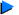# Factor Theorem အသံုးခ် ပုစာၦမ်ား

Example 2
Given that x2 + x - 6 is a factor of 2x4 + x3 - ax + bx + a + b - 1, find the value of a and b.x2 + x - 6 = (x + 3)(x + 2)

Let f(x) = 2x4 + x3 - ax2 + bx + a + b - 1
f(-3) = 2(-3)4 + (-3)3 - a(-3)2 - 3b + a + b - 1 = 0
134 - 8a - 2b = 0
4a + b = 67 --------(1)

f(2) = 2(2)4 + 23 - a(2)2 + 2b + a + b - 1 = 0

39 - 3a + 3b = 0
a - b = 13 --------(2)

(1) + (2) : 5a = 80

a = 16
when a = 16, b = 3

Example 3

Use the factor theorem to find the value of k for which (a + 2b) where a does not = 0 and b does not = 0, is a factor of a4 + 32b4 + a3b(k + 3).Solution

Let f(a) = a4 + 32b4 + a3b(k + 3)
f(-2b) = (-2b)4 + 32b4 + (-2b)3b(k + 3) = 0
48b4 - 8b4(k+ 3) = 0
8b4[6 - (k + 3)] = 0
8b4(3 - k) = 0
Since b does not = 0, 3 - k = 0
k = 3

Example 4
Determine the value of k for which x + 2 is a factor of (x + 1)7 + (2x + k)3.Solution

Let f(x) = (x + 1) + (2x + k)
f(-2) = (-2 + 1) + (-4 + k) = 0
(k - 4) - 1 = 0
(k - 4) = 1
k - 4 = 1
k = 5

Example 5
Given that f(x) = x3 - x2 - ax - b, where a and b are constants, has the factor x - 3 but has a remainder of 13x - 11 when divided by x + 4. Calculate the values of a and b.
Hence (i) factorise f(x) completely. (ii) Solve the equation 27x3 - 3ax = ax2 + b.Solution

f(3) = 0
33 - 32 - 3a - b = 0
3a + b = 18 --------(1)
f(-4) = (-4)3 - (-4)2 - a(-4) - b = 13(-4) - 11
4a - b = 17 --------(2)
sub (1) into (2): a = 5, b = 3
(i) Factorising.
f(x) = (x - 3)(x2 + 2x + 1)
= (x - 3)(x + 1)2
(ii) 27x3 - 9x2 - 3ax - b = 0
(3x)3 - (3x)2 - a(3x) - b = 0
Let y = 3x
y3 - y2 - ay - b = 0
(y - 3)(y + 1)2 = 0 [from (1)]
y = 3, y = -1
x = 1, x = -1/3

Example 6
Show that the expression x3 + (k-2) x2 + (k-7)x - 4 has a factor x+1 for all values of k .If the expression also has a factor x+2, find the value of k and the third factor.Solution

Let f(x) = x3 + (k-2) x2 + (k-7)x - 4
f(-1) =(-1)3 + (k-2)(-1)2 + (k-7)(-1) - 4 = -1+ k - 2 - k + 7 - 4 = 0
Therefore (x+1) is a factor of f(x).
x + 2 is a factor of f(x). -------(given)
Therefore f(-2)=0
(-2)3 + (k-2)(-2)2 + (k-7)(-2) - 4 = 0
-8 + 4k -8 - 2k +14 - 4 = 0
2k = 6
k = 3
Therefore f(x) = x3 + x2 - 4x - 4
Therefore (x + 1) (x + 2) = x2 + 3x + 2 is also a factor of f(x).Therefore the third factor of f(x) is x - 2.

Example 7
Given that kx3 + 2
x2 + 2x + 3 is a factor of kx3 - 2x + 9, have a common factor, what are the possible values of k?Solution
Let f(x) =
>kx3 + 2x2 + 2x +
3 and g(x) = kx3 - 2x + 9.
Let (x - c) be a common factor of both f(x) and g(x).Therefore ...
f(c) = 0
kc3 + 2c2 + 2c + 3 = 0 ---------(1)

g(c) = 0
kc3 - 2c + 9= 0 ---------(2)

Subtracting equation(2) from (1),we have
2c2 + 4c - 6 = 0
c2 + 2c - 3 = 0
(c + 3) (c - 1) =0
c = -3 or c = 1

Substituting c = -3 or c = 1 in equation (2), we get
k = 5/9 when c = -3 and
k = -7 when c = 1